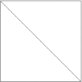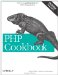# Recipe 17.1. Drawing Lines, Rectangles, and Polygons

#### 17.1.2. Problem

You want to draw a line, rectangle, or polygon. You also want to be able to control if the rectangle or polygon is open or filled in. For example, you want to be able to draw bar charts or create graphs of stock quotes.

#### 17.1.3. Solution

To draw a line, use ImageLine( ):

`ImageLine(\$image, \$x1, \$y1, \$x2, \$y2, \$color);`

To draw an open rectangle, use ImageRectangle( ):

`ImageRectangle(\$image, \$x1, \$y1, \$x2, \$y2, \$color);`

To draw a solid rectangle, use ImageFilledRectangle( ):

`ImageFilledRectangle(\$image, \$x1, \$y1, \$x2, \$y2, \$color);`

To draw an open polygon, use ImagePolygon( ):

`\$points = array(\$x1, \$y1, \$x2, \$y2, \$x3, \$y3); ImagePolygon(\$image, \$points, count(\$points)/2, \$color);`

To draw a filled polygon, use ImageFilledPolygon( ):

`\$points = array(\$x1, \$y1, \$x2, \$y2, \$x3, \$y3); ImageFilledPolygon(\$image, \$points, count(\$points)/2, \$color);`

#### 17.1.4. Discussion

The prototypes for all five functions in the Solution are similar. The first parameter is the canvas to draw on. The next set of parameters are the x and y coordinates to specify where GD should draw the shape. In ImageLine( ), the four coordinates are the endpoints of the line, and in ImageRectangle( ), they're the opposite corners of the rectangle. For example, ImageLine(\$image, 0, 0, 100, 100, \$color) produces a diagonal line. Passing the same parameters to ImageRectangle( ) produces a rectangle with corners at (0,0), (100,0), (0,100), and (100,100). Both shapes are shown in Figure 17-2.

##### A diagonal line and a squareThe ImagePolygon( ) function is slightly different because it can accept a variable number of vertices. Therefore, the second parameter is an array of x and y coordinates. The function starts at the first set of points and draws lines from vertex to vertex before finally completing the figure by connecting back to the original point. You must have a minimum of three vertices in your polygon (for a total of six elements in the array). The third parameter is the number of vertices in the shape; since that's always half of the number of elements in the array of points, a flexible value for this is count(\$points) / 2 because it allows you to update the array of vertices without breaking the call to ImageLine( ).

Last, all the functions take a final parameter that specifies the drawing color. This is usually a value returned from ImageColorAllocate( ) but can also be the constants IMG_COLOR_STYLED or IMG_COLOR_STYLEDBRUSHED, if you want to draw nonsolid lines, as discussed in Recipe 17.3.

These functions all draw open shapes. To get GD to fill the region with the drawing color, use ImageFilledRectangle( ) and ImageFilledPolygon( ) with the identical set of arguments as their unfilled cousins.

Recipe 17.2 for more on drawing other types of shapes; Recipe 17.3 for more on drawing with styles and brushes; documentation on ImageLine( ) at http://www.php.net/imageline, ImageRectangle( ) at http://www.php.net/imagerectangle, ImagePolygon( ) at http://www.php.net/imagepolygon, and ImageColorAllocate( ) at http://www.php.net/imagecolorallocate.PHP Cookbook: Solutions and Examples for PHP Programmers
ISBN: 0596101015
EAN: 2147483647
Year: 2006
Pages: 445

Similar book on Amazon